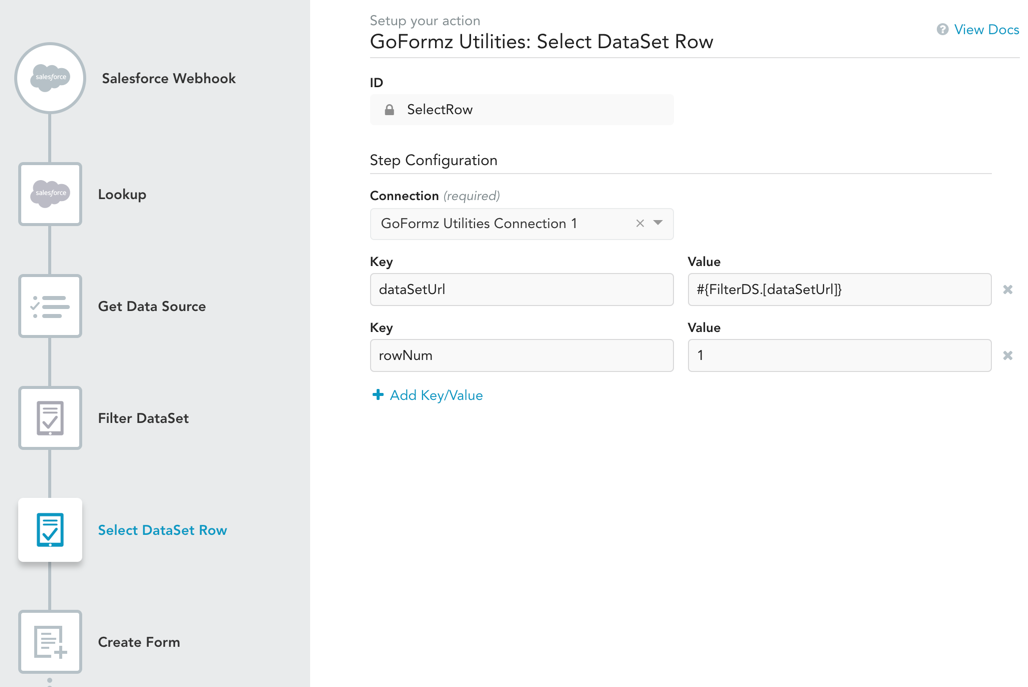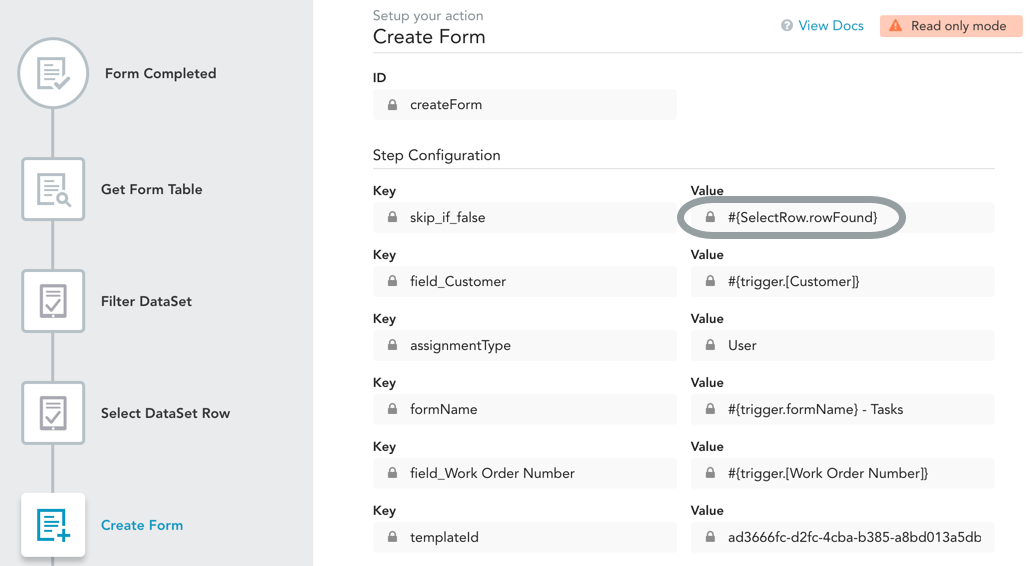# Select DataSet Row

Access column values for a selected DataSet file row. This is currently the only action that outputs individual values from a DataSet in a way that can be used by other actions.

# Inputs

Input

Value

`dataSetUrl`

Required

The URL of the DataSet JSON file from which you want to extract row values.

`rowNum`

Required

The row number that you want to access from `dataSetUrl`.

# Outputs

Key

Value

`rowFound`

`true` if the row was found, `false` otherwise. This will only be `false` if the `rowNum` input is greater than the total number of rows in your DataSet file.

`field_<column_name>`

The contents of each column in the selected row. For example, if your DataSet has columns named "Contact Name", "Email" and "Phone", then there will be three field outputs — `field_Contact Name`, `field_Email` and `field_Phone`.

# Usage

Extracting individual values from a DataSet can be handy in a number of situations. For example, you may want to use values from a (GoFormz or external) data source to fill in a form.

There are a number of different ways to use this action. For instance:

• If you know the row number that you want to extract from the DataSet, you can use this action directly, setting `rowNum` to the row number you want.
• If you want to select a row based on certain properties, you can use Filter DataSet first to isolate the row you want from the DataSet, and then use Select DataSet Row with `rowNum=1` to extract the row values. This use case shown in the image below. This shows the Select DataSet Row step of the same example that we review in the Filter DataSet documentation. In this scenario, we find a customer in a GoFormz Data Source using the Get Data Source and Filter DataSet actions; then use Select DataSet Row to extract the column values from the one row of the filtered DataSet (`#{FilterDS.[dataSetUrl]}`); then use those column values in Create Form to fill form fields.• Another use case for this action is to simply check if a DataSet is empty. You can do this by using `rowNum=1` and then checking to see if `rowFound=false`. In the example below, we have a Create Form action that only executes if a DataSet is not empty. We do this by setting skip_if_false to the `rowFound` output of the Select DataSet Row step.